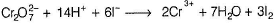Courses

# Test: Oxides & Oxo Anions Of Cr And Mn

## 25 Questions MCQ Test Chemistry for JEE | Test: Oxides & Oxo Anions Of Cr And Mn

Description
This mock test of Test: Oxides & Oxo Anions Of Cr And Mn for JEE helps you for every JEE entrance exam. This contains 25 Multiple Choice Questions for JEE Test: Oxides & Oxo Anions Of Cr And Mn (mcq) to study with solutions a complete question bank. The solved questions answers in this Test: Oxides & Oxo Anions Of Cr And Mn quiz give you a good mix of easy questions and tough questions. JEE students definitely take this Test: Oxides & Oxo Anions Of Cr And Mn exercise for a better result in the exam. You can find other Test: Oxides & Oxo Anions Of Cr And Mn extra questions, long questions & short questions for JEE on EduRev as well by searching above.
QUESTION: 1

### Only One Option Correct Type Direction (Q. Nos. 1-10) This section contains 10 multiple choice questions. Each question has four choices (a), (b), (c) and (d), out of which ONLY ONE is correct. Q. Which is most basic oxide of chromium?

Solution:

Oxide having lower oxidation number is basic and as oxidation number increase, acidic nature increases.

QUESTION: 2

### The basic character of the transition metal monoxides follows the order (Atomic Number, Ti = 22, V = 23, Cr = 24, Fe = 26)

Solution:

TiO > VO > CrO > FeO with the decrease in size of metal atom from Ti to Fe, the basic character of their monoxides decreases.

QUESTION: 3

### MnO4-  + S2- + H+ → Mn2+ +S + H2O When this equation is balanced, the total number of coefficients on the left hand side are

Solution: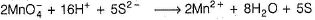QUESTION: 4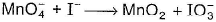when this equation is balanced, the num ber of OF- ions added

Solution: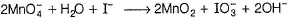QUESTION: 5

One mole of each KMnO4 and K2Cr2O7 can oxidise ........... moles of ferrous ion.

Solution:

One equivalent of KMnO4 = One equivalent of K2Cr2O7 = One equivalent of Fe2+ ion or 1/5 moles of KMnO4 = 1/6 moles of K2Cr2O7 = One mole of Fe2+ ion.
Hence, one mole of KMnO4 reacts with 5 moles of Fe2+ ion and one mole of K2Cr2O7 reacts with 6 moles of Fe2+ ion.

QUESTION: 6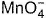is of intense pink colour, though Mn is in (+7) oxidation state. It is due to

Solution:

This is called ligand to metal charge transfer phenomenon.

QUESTION: 7

Which of the following is incorrect statement?

Solution:

The green manganate is paramagnetic with one unpaired electron but the permanganate is diamagnetic.

QUESTION: 8

Pyrolusite in MnO2 is used to prepare KMnO4. Steps are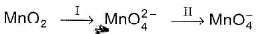Here, I and II are

Solution: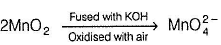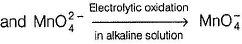QUESTION: 9

Which is the correct statem ent about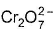structure?

Solution:

The correct answer is Option A.
In a dichromate dianion structure, the negative charge can be on any O attached to Cr, therefore all Cr - O are equivalent. Also have Cr - O - Cr bond.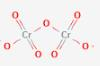QUESTION: 10

Out of Cr (VI) as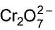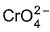, which is better oxidising agent?

Solution:+ 4H2O + 3e- → Cr(OH)3 + 5OH-, E° = - 0.13 V+ 14H+ + 6e- → 2Cr3+ + 7H2O , E° = 1.33 V

*Multiple options can be correct
QUESTION: 11

One or More than One Options Correct Type

Direction (Q. Nos. 11-15) This section contains 5 multiple choice questions. Each question has four choices (a), (b), (c) and (d), out of which ONE or MORE THAN ONE are correct.

Q.

Which of the following statem ents is correct when a mixture of NaCI and K2Cr2O7 is gently warmed with cone. H2SO4?

Solution: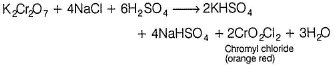*Multiple options can be correct
QUESTION: 12

Amphoteric oxides in the following is/are

Solution:

Mn2O7 is acidic while others are amphoteric.

*Multiple options can be correct
QUESTION: 13

Which of the following on heating produce oxygen gas?

Solution: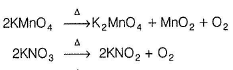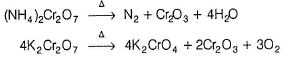QUESTION: 14

Which of the following statements is not true?

Solution:

Potassium dichromate is used as a primary standard in volumetric analysis.

*Multiple options can be correct
QUESTION: 15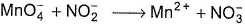when this redox reaction is balanced in acidic medium, we get

Solution: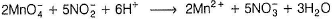QUESTION: 16

Comprehension Type

Direction (Q. Nos. 16 and 17) This section contains a paragraph, describing theory, experiments, data, etc. Two questions related to the paragraph have been given. Each question has only one correct answer among the four given options (a), (b), (c) and (d).

Passage

Oxides are generally formed by the reaction of metals with oxygen at high temperatures. All the metals except scandium form MO oxides which are ionic. The highest oxidation number in the oxides, coincides with the group number and is attained in Sc2O3 to Mn2O7. Beyond 7th group, higher oxides coinciding with the group number do not form. As the oxidation number of metal increases covalent character and acidity increases. Mn2O7, CrO3 are acidic and their lower oxides are basic and amphoteric. Stability of higher oxides increases down the group.

Q.

Which of the following reacts with both acids and bases?

Solution:

ZnO and V2O5 are am photeric oxides.

QUESTION: 17

Oxides are generally formed by the reaction of metals with oxygen at high temperatures. All the metals except scandium form MO oxides which are ionic. The highest oxidation number in the oxides, coincides with the group number and is attained in Sc2O3 to Mn2O7. Beyond 7th group, higher oxides coinciding with the group number do not form. As the oxidation number of metal increases covalent character and acidity increases. Mn2O7, CrO3 are acidic and their lower oxides are basic and amphoteric. Stability of higher oxides increases down the group.

Q.

Which of the following undergo disproportionation?

Solution: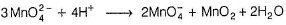QUESTION: 18

Matching List Type

Direction (Q. Nos. 18 and 19) Choices for the correct combination of elements from Column I and Column II are given as options (a), (b), (c) and (d), out of which one is correct.

Q.

Match the Column I with Column II and mark the correct option from the codes given below.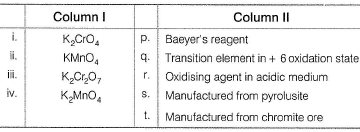Solution:

(i) → (q,t) (ii) → (p.r) (iii) → (q.r.t) (iv) → (q,s)

QUESTION: 19

Match the Column I with Column II and mark the correct option from the codes given below.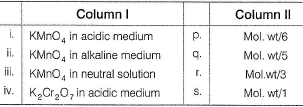Solution:

(i) → (q) (ii) → (s) (iii) → (r) (iv) → (p)

(i) In acidic medium,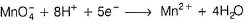Hence, = M/5
(ii) In strongly alkaline medium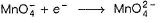Hence, = M/1
(iii) In neutral or weakly basic medium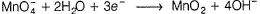Hence, = M/3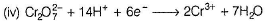Hence, equivalent weight is M/6.

*Answer can only contain numeric values
QUESTION: 20

One Integer Value Correct Type

Direction (Q. Nos. 20-23) This section contains 4 questions. When worked out will result in an integer from 0 to 9 (both inclusive

Q.

The number of moles of FeC2O4 that reduces two moles of KMnO4 in acidic medium are

Solution: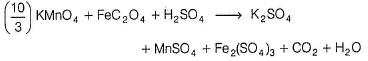Balanced equation,
6KMnO4 + 10FeC2O4 + 24H2SO4 → 3K2SO4 + 6MnSO4 + 5Fe2(SO4)3 + 20 CO2 + 24 H2
Thus, 6 moles of KMnO4 react with 10FeC2O4.
Hence, 2 moles of KMnO4 react with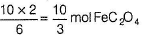*Answer can only contain numeric values
QUESTION: 21

50 cc of 0.04 M K2Cr2O7 in acidic medium oxidises a sample of H2S gas to sulphur. Volume of 0.03 M KMnO4 required to oxidise the same amount of H2S gas to sulphur in acidic medium is 10 x x. Here, the value of x is

Solution:

Milliequivalents of K2Cr2O7 reacted with H2S (N1V1) = miliiequivalents of KMnO4 reacted with H2S(N2V2), i.e. milliequivalent of KMnO4 = milliequivalent of H2S Therefore, 50 x 0.04 x 6 = V2 x 0.03 x 5
or V2 = 80 mL

*Answer can only contain numeric values
QUESTION: 22

The number of sigma bonds in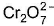ion are

Solution: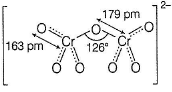*Answer can only contain numeric values
QUESTION: 23

If molarity of K2Cr2O7 solution is 0.5 M, its normality value is

Solution:

Normality = molarity x number of electrons gained per mole of dichromate in a redox reaction. Hence, Normality = Molarity x 6

QUESTION: 24

Statement Type

Direction (Q. Nos. 24 and 25) This section is based on Statement I and Statement II. Select the correct answer from the codes given below.

Q.

Statement I ; When a solution of potassium chromate is treated with an excess of dilute nitric acid chromate undergoes oxidation.

Statement II : Dichromate ions are produced in above process.

Solution: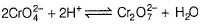QUESTION: 25

Statement I : The oxidation state of chromium in the final product formed by the reaction between Kl and acidified potassium dichromate solution has green colour.

Statement II : The product has Cr3+ ions.

Solution: